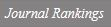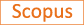View : 362 Download: 0

Simple valuation ideals of order 3 in two-dimensional regular local rings

Title
Simple valuation ideals of order 3 in two-dimensional regular local rings
Authors
Noh S.
Ewha Authors
SCOPUS Author ID
노선숙Issue Date
2008
Journal Title
Communications of the Korean Mathematical Society
ISSN
1225-1763Citation
Communications of the Korean Mathematical Society vol. 23, no. 4, pp. 511 - 528
Indexed
SCOPUS; KCIDocument Type
Article
Abstract
Let (R,m) be a 2-dimensional regular local ring with algebraically closed residue field R/m. Let K be the quotient field of R and v be a prime divisor of R, i.e., a valuation of K which is birationally dominating R and residually transcendental over R. Zariski showed that there are finitely many simple v-ideals m = P0 ⊃ P1 ⊃ ... ⊃ Pt = P and all the other v-ideals are uniquely factored into a product of those simple ones . Lipman further showed that the predecessor of the smallest simple v-ideal P is either simple or the product of two simple v-ideals. The simple integrally closed ideal P is said to be free for the former and satellite for the later. In this paper we describe the sequence of simple v-ideals when P is satellite of order 3 in terms of the invariant bv =

v(x) - v(y)

, where v is the prime divisor associated to P and m = (x, y). Denote bv by b and let b = 3k + 1 for k = 0, 1, 2. Let ni be the number of nonmaximal simple v-ideals of order i for i = 1, 2, 3. We show that the numbers nv = (n1, n2, n3) = ([b+1/3], 1, 1) and that the rank of P is = [b+7/3] = k+3. We then describe all the v-ideals from • to P as products of those simple v-ideals. In particular, we find the conductor ideal and the v-predecessor of the given ideal P in cases of b = 1, 2 and for b = 3k + 1, 3k + 2, 3k for k ≥ 1. We also find the value semigroup v(R) of a satellite simple valuation ideal P of order 3 in terms of bv. © 2008 The Korean Mathematical Society.
DOI
10.4134/CKMS.2008.23.4.511
Appears in Collections:
사범대학 > 수학교육과 > Journal papers
Files in This Item:
There are no files associated with this item.
Export
RIS (EndNote)
XLS (Excel)
XMLItems in DSpace are protected by copyright, with all rights reserved, unless otherwise indicated.